# Most Powerful Gemstones and Crystals for Scorpios - Psychic Readings by Ronn

Nov. 1, 2018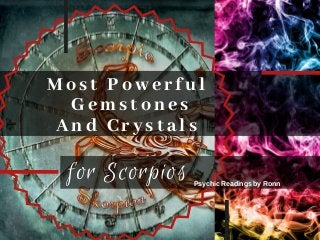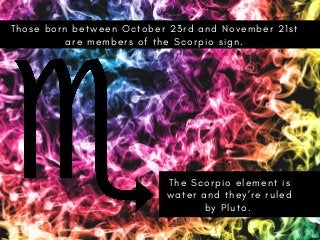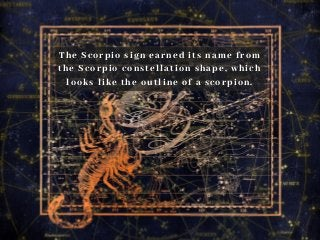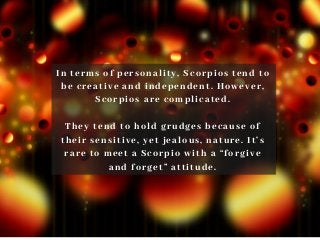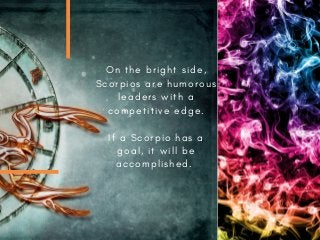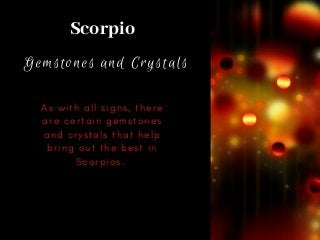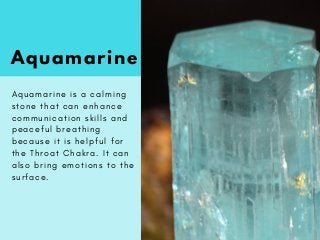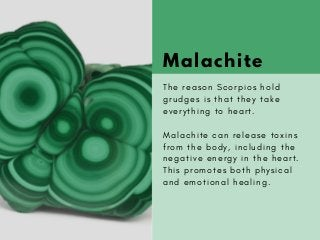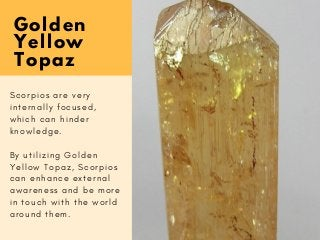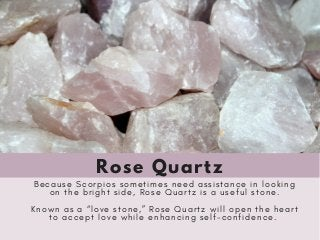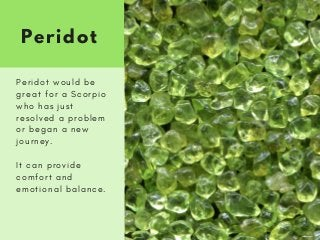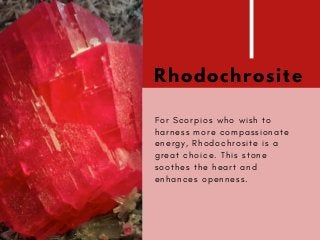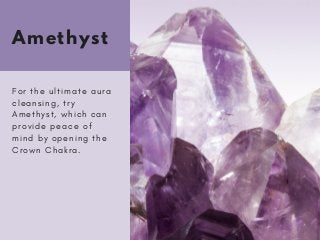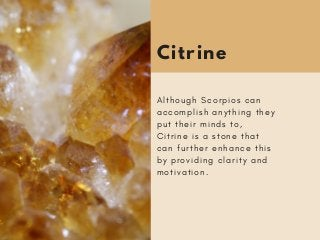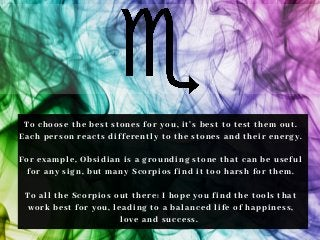1 of 15

### Most Powerful Gemstones and Crystals for Scorpios - Psychic Readings by Ronn

• 1. Most Powerful  Gemstones And Crystals for Scorpios Psychic Readings by Ronn
• 2. T h o s e b o r n b e t w e e n O c t o b e r 2 3 r d a n d N o v e m b e r 2 1 s t a r e m e m b e r s o f t h e S c o r p i o s i g n . T h e S c o r p i o e l e m e n t i s w a t e r a n d t h e y ’ r e r u l e d b y P l u t o .
• 3. The Scorpio sign earned its name from the Scorpio constellation shape, which looks like the outline of a scorpion.
• 4. In terms of personality, Scorpios tend to be creative and independent. However, Scorpios are complicated. They tend to hold grudges because of their sensitive, yet jealous, nature. It’s rare to meet a Scorpio with a “forgive and forget” attitude.
• 5. O n t h e b r i g h t s i d e , S c o r p i o s a r e h u m o r o u s l e a d e r s w i t h a c o m p e t i t i v e e d g e . I f a S c o r p i o h a s a g o a l , i t w i l l b e a c c o m p l i s h e d .
• 6. Gemstones and Crystals A s w i t h a l l s i g n s , t h e r e a r e c e r t a i n g e m s t o n e s a n d c r y s t a l s t h a t h e l p b r i n g o u t t h e b e s t i n S c o r p i o s .   Scorpio
• 7. Aquamarine A q u a m a r i n e i s a c a l m i n g s t o n e t h a t c a n e n h a n c e c o m m u n i c a t i o n s k i l l s a n d p e a c e f u l b r e a t h i n g b e c a u s e i t i s h e l p f u l f o r t h e T h r o a t C h a k r a . I t c a n a l s o b r i n g e m o t i o n s t o t h e s u r f a c e .
• 8. Malachite T h e r e a s o n S c o r p i o s h o l d g r u d g e s i s t h a t t h e y t a k e e v e r y t h i n g t o h e a r t . M a l a c h i t e c a n r e l e a s e t o x i n s f r o m t h e b o d y , i n c l u d i n g t h e n e g a t i v e e n e r g y i n t h e h e a r t . T h i s p r o m o t e s b o t h p h y s i c a l a n d e m o t i o n a l h e a l i n g .
• 9. Golden Yellow Topaz S c o r p i o s a r e v e r y i n t e r n a l l y f o c u s e d , w h i c h c a n h i n d e r k n o w l e d g e . B y u t i l i z i n g G o l d e n Y e l l o w T o p a z , S c o r p i o s c a n e n h a n c e e x t e r n a l a w a r e n e s s a n d b e m o r e i n t o u c h w i t h t h e w o r l d a r o u n d t h e m .
• 10. Rose Quartz B e c a u s e S c o r p i o s s o m e t i m e s n e e d a s s i s t a n c e i n l o o k i n g o n t h e b r i g h t s i d e , R o s e Q u a r t z i s a u s e f u l s t o n e . K n o w n a s a “ l o v e s t o n e , ” R o s e Q u a r t z w i l l o p e n t h e h e a r t t o a c c e p t l o v e w h i l e e n h a n c i n g s e l f - c o n f i d e n c e .
• 11. Peridot P e r i d o t w o u l d b e g r e a t f o r a S c o r p i o w h o h a s j u s t r e s o l v e d a p r o b l e m o r b e g a n a n e w j o u r n e y . I t c a n p r o v i d e c o m f o r t a n d e m o t i o n a l b a l a n c e .
• 12. Rhodochrosite F o r S c o r p i o s w h o w i s h t o h a r n e s s m o r e c o m p a s s i o n a t e e n e r g y , R h o d o c h r o s i t e i s a g r e a t c h o i c e . T h i s s t o n e s o o t h e s t h e h e a r t a n d e n h a n c e s o p e n n e s s .
• 13. Amethyst F o r t h e u l t i m a t e a u r a c l e a n s i n g , t r y A m e t h y s t , w h i c h c a n p r o v i d e p e a c e o f m i n d b y o p e n i n g t h e C r o w n C h a k r a .
• 14. Citrine A l t h o u g h S c o r p i o s c a n a c c o m p l i s h a n y t h i n g t h e y p u t t h e i r m i n d s t o , C i t r i n e i s a s t o n e t h a t c a n f u r t h e r e n h a n c e t h i s b y p r o v i d i n g c l a r i t y a n d m o t i v a t i o n .
• 15. To choose the best stones for you, it’s best to test them out. Each person reacts differently to the stones and their energy. For example, Obsidian is a grounding stone that can be useful for any sign, but many Scorpios find it too harsh for them. To all the Scorpios out there: I hope you find the tools that work best for you, leading to a balanced life of happiness, love and success.